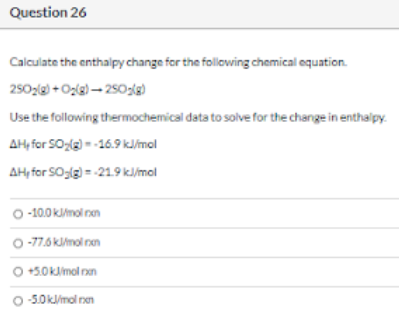# Calculate the enthalpy change for the following chemical equation. 2SO2(g) + O2(g) → 2SO3(g) Use the following thermochemical data to solve for the change in enthalpy. ΔHf for SO2(g) = -16.9 kJ/mol ΔHf for SO3(g) = -21.9 kJ/mol a) -10.0 kJ/mol rxn b) -77.6 kJ/mol rxn c) +5.0 kJ/mol rxn d) -5.0 kJ/mol rxn